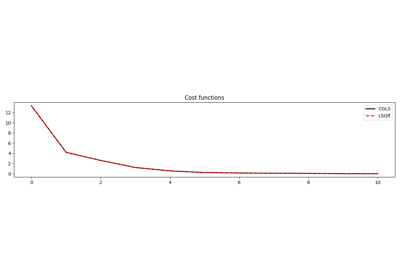# pylops.optimization.basic.cgls#

pylops.optimization.basic.cgls(Op, y, x0=None, niter=10, damp=0.0, tol=0.0001, show=False, itershow=(10, 10, 10), callback=None)[source]#

Solve an overdetermined system of equations given an operator Op and data y using conjugate gradient iterations.

Parameters
Oppylops.LinearOperator

Operator to invert of size $$[N \times M]$$

ynp.ndarray

Data of size $$[N \times 1]$$

x0np.ndarray, optional

Initial guess

niterint, optional

Number of iterations

dampfloat, optional

Damping coefficient

tolfloat, optional

Tolerance on residual norm

showbool, optional

Display iterations log

itershowtuple, optional

Display set log for the first N1 steps, last N2 steps, and every N3 steps in between where N1, N2, N3 are the three element of the list.

callbackcallable, optional

Function with signature (callback(x)) to call after each iteration where x is the current model vector

Returns
xnp.ndarray

Estimated model of size $$[M \times 1]$$

istopint

Gives the reason for termination

1 means $$\mathbf{x}$$ is an approximate solution to $$\mathbf{y} = \mathbf{Op}\,\mathbf{x}$$

2 means $$\mathbf{x}$$ approximately solves the least-squares problem

iitint

Iteration number upon termination

r1normfloat

$$||\mathbf{r}||_2$$, where $$\mathbf{r} = \mathbf{y} - \mathbf{Op}\,\mathbf{x}$$

r2normfloat

$$\sqrt{\mathbf{r}^T\mathbf{r} + \epsilon^2 \mathbf{x}^T\mathbf{x}}$$. Equal to r1norm if $$\epsilon=0$$

costnumpy.ndarray, optional

History of r1norm through iterations

Notes

## Examples using pylops.optimization.basic.cgls#CGLS and LSQR Solvers

CGLS and LSQR Solvers